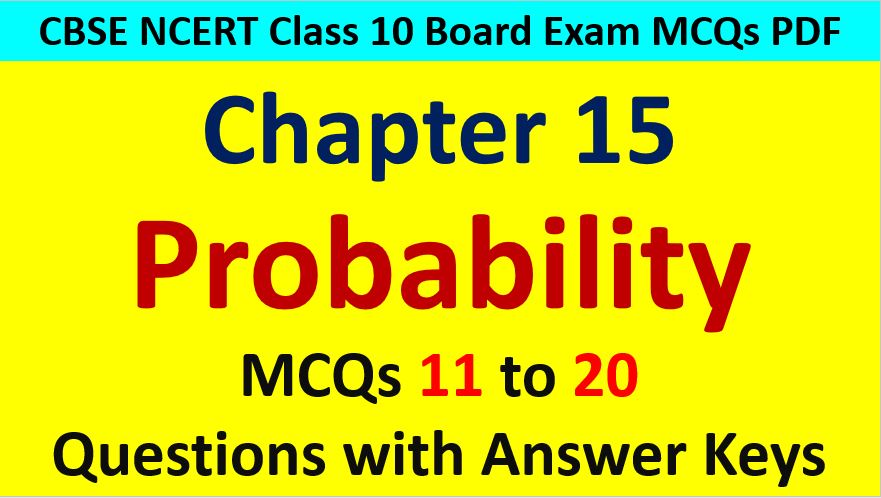Saturday, December 4, 2021
Home > CBSE Class 10 > Probability Class 10 Maths MCQ Questions with Answer Keys

# Probability Class 10 Maths MCQ Questions with Answer KeysHi students, Welcome to AMBiPi (Amans Maths Blogs). In this article, you will get Probability Class 10 Maths MCQ Questions with Answer Keys. You can download this PDF and save it in your mobile device or laptop etc.

Probability MCQ Questions for Class 10 Question No 11:

The probability that a number selected at random from the numbers 1, 2, 3, …., 15 is a multiple of 4 is

Option A : 4/15

Option B : 2/15

Option C : 1/15

Option D : 1/5

Option D : 1/5

Probability MCQ Questions for Class 10 Question No 12:

Two coins are tossed simultaneously. The probability of getting at most one head is

Option A : 1/4

Option B : 1/2

Option C : 2/3

Option D : 3/4

Option D : 3/4

Probability MCQ Questions for Class 10 Question No 13:

If an event cannot occur, then its probability is

Option A : 1

Option B : 0.1

Option C : 3%

Option D : 0

Option D : 0

Probability MCQ Questions for Class 10 Question No 14:

Which of the following cannot be the probability of an event?

Option A : 1/3

Option B : 0.1

Option C : 3%

Option D : 17/16

Option D : 17/16

Probability MCQ Questions for Class 10 Question No 15:

An event is very unlikely to happen. Its probability is closest to

Option A : 0.0001

Option B : 0.001

Option C : 0.01

Option D : 0.1

Option A : 0.0001

Probability MCQ Questions for Class 10 Question No 16:

If the probability of an event is p, then the probability is its complementary event will be

Option A : p – 1

Option B : p

Option C : 1 – p

Option D : 1 – 1/p

Option C : 1 – p

Probability MCQ Questions for Class 10 Question No 17:

The probability expressed as a percentage of a particular occurrence can never be

Option A : less than 100

Option B : less than 0

Option C : greater than 1

Option D : anything but a whole number

Option B : less than 0

Probability MCQ Questions for Class 10 Question No 18:

If P(A) denotes the probability of an event A, then

Option A : P(A) < 0

Option B : P(A) > 0

Option C : 0 ≤ P(A) ≤ 1

Option D : -1 ≤ P(A) ≤ 1

Option D : -1 ≤ P(A) ≤ 1

Probability MCQ Questions for Class 10 Question No 19:

If a card is selected from a deck of 52 cards, then the probability of its being a red face card is

Option A : 3/26

Option B : 3/16

Option C : 2/13

Option D : 1/2

Option A : 3/26

Probability MCQ Questions for Class 10 Question No 20:

A card is drawn from a deck of 52 cards. The event E is that card is not an ace of hearts. The number of outcomes favorable to E is

Option A : 4

Option B : 13

Option C : 48

Option D : 51

Option D : 51

CBSE Class 10 Maths Chapterwise MCQs
1 : Real Numbers MCQ
| 1 to 10 | 11 to 20 | 21 to 30 | 31 to 40 | 41 to 50
2 : Polynomials MCQ
| 1 to 10 | 11 to 20 | 21 to 30 | 31 to 40 | 41 to 50
3 : Linear Equations MCQ
| 1 to 10 | 11 to 20 | 21 to 30 | 31 to 40 | 41 to 50
| 1 to 10 | 11 to 20 | 21 to 30 | 31 to 40 | 41 to 50
5 : Arithmetic Progression MCQ
| 1 to 10 | 11 to 20 | 21 to 30 | 31 to 40 | 41 to 50
6 : Triangles MCQ
| 1 to 10 | 11 to 20 | 21 to 30 | 31 to 40 | 41 to 50
7 : Coordinate Geometry MCQ
| 1 to 10 | 11 to 20 | 21 to 30 | 31 to 40 | 41 to 50
8 : Introduction to Trigonometry MCQ
| 1 to 10 | 11 to 20 | 21 to 30 | 31 to 40 | 41 to 50
9 : Application of Trigonometry MCQ
| 1 to 10 | 11 to 20 | 21 to 30 | 31 to 40 | 41 to 50
10 : Circles MCQ
| 1 to 10 | 11 to 20 | 21 to 30 | 31 to 40 | 41 to 50
11 : Constructions MCQ
| 1 to 10 | 11 to 20
12 : Area Related to Circles MCQ
| 1 to 10 | 11 to 20 | 21 to 30 | 31 to 40 | 41 to 50
13 : Surface Area & Volume MCQ
| 1 to 10 | 11 to 20 | 21 to 30 | 31 to 40 | 41 to 50
14 : Statistics MCQ
| 1 to 10 | 11 to 20 | 21 to 30 | 31 to 40 | 41 to 50
15 : Probability MCQ
| 1 to 10 | 11 to 20 | 21 to 30 | 31 to 40 | 41 to 50
16 : Previous Year Maths MCQs from CBSE Board Class 10
| 1 to 100
AMBiPi
error: Content is protected !!